# Battery Cell Characterization for Electric Vehicles

This example uses the test method defined in  to characterize a battery cell for electric vehicle applications. First you generate a high pulse power characterization (HPPC) by using Simscape™ Battery™ blocks and an in-built function to derive the battery cell parameters. Then you compare the model to the original battery model under typical drive-profile loading conditions.

### Battery HPPC Test Data

A typical HPPC data is a set of discharge-charge pulses, applied to a battery at different state of charge (SOC) and at a given temperature. Typically, the test equipment is fully charged to undergo these pulse tests. At the end of every sequence, the SOC is discharged by a third of the C-rate. A long rest time of one hour is recommended for the cells to relax after every sequence of discharge-charge pulses. This process continues until it covers all points of interest in the SOC range.

This figure shows a typical discharge-charge profile. For more information, see .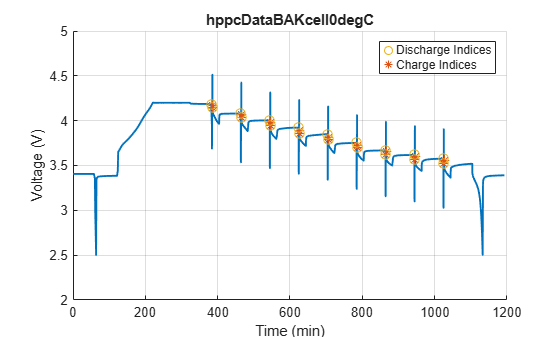### Parameter Estimation Method

The Battery (Table-Based) block in Simscape Battery uses the equivalent circuit modelling approach. You can capture different physical phenomena of a cell by connecting multiple RC pairs in series. In Battery (Table-Based) block, you can select up to five RC pairs. You can derive the value of the resistance and time constant parameters from the HPPC test data.

The voltage response of a battery cell is equal to:

`$\mathit{V}={\mathit{V}}_{0}-\mathit{I}×{\mathit{R}}_{\mathit{o}}-\mathit{I}×\left(\sum {\mathit{R}}_{\mathit{i}}\left(1-\mathrm{exp}\left(-\frac{\mathit{t}}{{\tau }_{\mathit{i}}}\right)\right)\right),$`

where

• ${\mathit{V}}_{0}$ is the cell open circuit potential.

• ${\mathit{R}}_{\mathit{o}}$ is the cell ohmic resistance.

• ${\mathit{R}}_{\mathit{i}}\text{\hspace{0.17em}}\mathrm{and}\text{\hspace{0.17em}}{\tau }_{\mathit{i}}$ are the cell i-th RC pair resistance and time constant values.

• $\mathit{I}$ is the current passing through the cell.

All parameters are a function of the SOC and cell temperature. Since HPPC tests are typically performed at constant temperatures, you can ignore the temperature dependence in the parameter estimation. The ohmic resistance is estimated from the sudden voltage change during discharge or charge pulses (V1 to V2 or V5 to V6, in the figure above). The RC pairs are fit based on voltage relaxation profile just after the discharge or charge pulses.

The `ParameterEstimationLUTbattery` function estimates the battery parameters and:

1. Takes the HPPC profile over entire SOC range as input.

2. Determines all the pulse location and the points V1 - V9 in the above figure.

3. Calculates the ohmic resistance value.

4. Fits the RC parameters by using either MATLAB `fminsearch` or the Curve Fitting Toolbox™ (fminsearch or curvefit).

5. Calculates the cell open circuit potential (point V1, at a given SOC point, in the figure above).

6. Outputs all parameters to a workspace variable.

### Generate Synthetic Test Data

Run the `CellCharacterizationHPPC` SLX file to generate the current and voltage data for the selected cell.The HPPC Profile MATLAB function defines the discharge-charge protocols and the test method.

`hppcSim = sim('CellCharacterizationHPPC.slx');`

The `hppcSim` workspace variable contains the current and voltage data for the HPPC profile used for battery cell parameter estimation.

### Fit Parameters to Test Data

Load the HPPC data and plot the voltage and current values.

```hppcTest = hppcSim.batteryHPPC_profile.extractTimetable; time = seconds(hppcTest.Time); current = hppcTest.current; voltage = hppcTest.voltage; figure('Name','HPPC data - current pulses') plot(time,current); xlabel('Time (s)');ylabel('Current (A)')``````figure('Name','HPPC data - voltage response') plot(time,voltage); xlabel('Time (s)');ylabel('Voltage (V)')```Define the cell capacity (Ahr) as during the HPPC tests and the initial SOC (0-1).

```cellCapacity = 27; cellInitialSOC = 1; cell_prop = [cellCapacity; cellInitialSOC];```

Define the pulse current magnitudes, in Amperes.

```maxDischargeCurr = 81; maxChargeCurr = 61; constCurrSweepSOC = 9;```

The `ParameterEstimationLUTbattery` function detects a pulse (sudden change in current) based on the value you specify for the `toleranceVal` variable. If the function detects a sudden change in discharge current, it compares this discharge current to the value of the `maxDischargeCurr` variable. If their difference is within the value of the `toleranceVal` variable, the function identifies the pulse. This process also applies to the detection of the charge pulse (`maxChargeCurr`) and the SOC sweep (`constCurrSweepSOC`).

```toleranceVal = 1; hppc_protocol = [maxDischargeCurr;... maxChargeCurr;... constCurrSweepSOC;... toleranceVal];```

Define the number of RC pairs to consider and the initial guess for resistance and the time constant values.

```numRCpairs = 1; initialGuess_RC = [1e-3 20]; % [R1, Tau1, R2, Tau2 ....] result=batt_BatteryCellCharacterization.ParameterEstimationLUTbattery(... [time, current, voltage],... cell_prop,... hppc_protocol,... numRCpairs,... initialGuess_RC,... "fminsearch");```
```Read input data *** Number of discharge pulses =20 *** Number of charge pulses =20 *** Number of SOC sweep pulses =19 Extracted pulse data from input data Calculated ohmic resistance *** Calculated RC parameters for discharge *** Calculated RC parameters for charge *** Calculated rmse for the fit Calculated RC parameters Completed OCV data extraction ```
```% To use curvefit toolbox for data fit, type "curvefit" % instead of "fminsearch". The curvefit function requires % the Curve Fitting Toolbox license.```

To check if the function identified the correct pulses, at a MATLAB Command Window, enter:

`plotAndVerifyPulseData(result);`To verify the fit, at a MATLAB Command Window, enter:

```fitDataEverySOCval = 0.001; fitDataForSOCpts = 0:fitDataEverySOCval:1; verifyDataFit(result,fitDataEverySOCval,1);```To save the parameters, enter:

```cellParameters = exportResultsForLib(result,... fitDataForSOCpts);```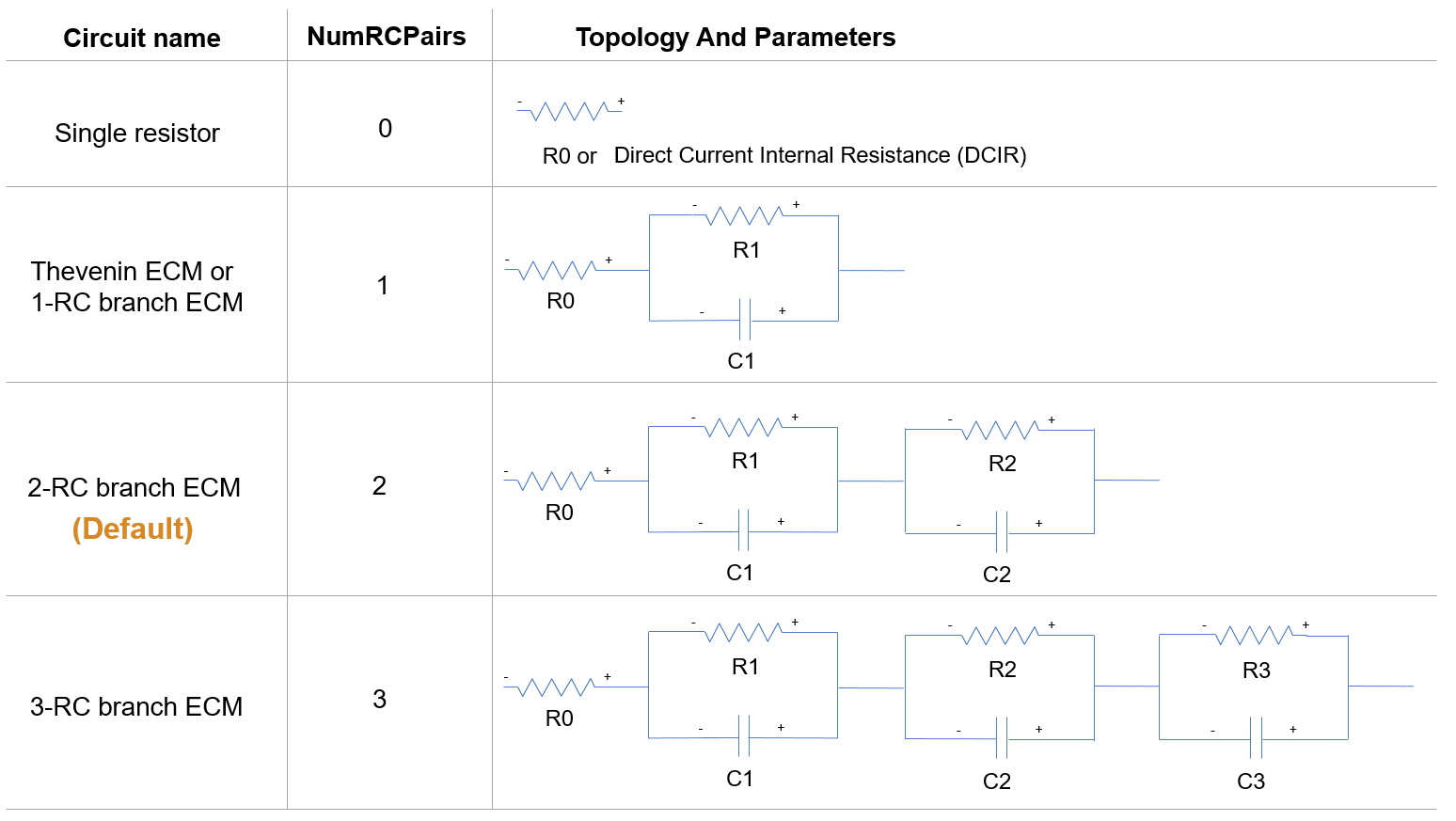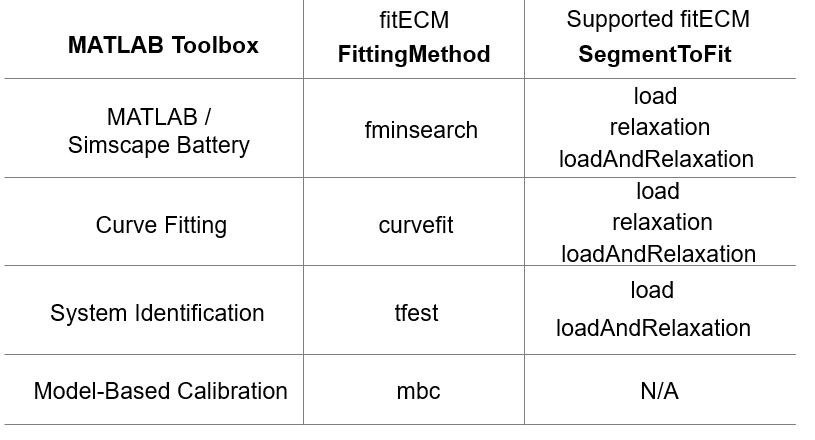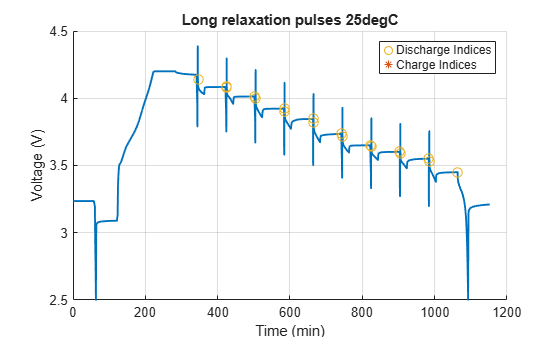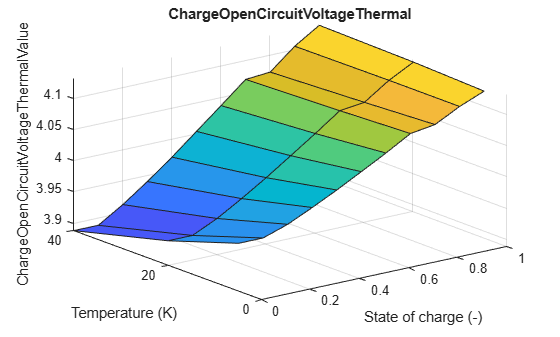To save the generated parameters in a file, at the MATLAB Command Window, run:

```save batt_BatteryCellCharacterizationResults.mat cellParameters ```

This example uses parameters stored in `batt_BatteryCellCharacterizationResults` MAT file to verify the accuracy of the fit. If the estimated parameters do not look reasonable, try fitting them with more RC pairs or try a different initial guess.

### Verify Parameters with Drive Profiles

A large battery pack for electric vehicle (EV) uses the battery cell that you just parameterized. The `CellCharacterizationVerify.slx` model uses a drive profile to compare the parameterized cell against the original cell.A typical load profile for a large battery pack (EV) is:

```driveProfile = load('batt_BatteryCellCharacterizationForBEV_Ivst.mat'); maxCurrentPack = max(driveProfile.ans.Data)```
```maxCurrentPack = 89.5372 ```
`minCurrentPack = min(driveProfile.ans.Data)`
```minCurrentPack = -55.2423 ```
```figure('Name','Drive profile'); plot(driveProfile.ans.Time,driveProfile.ans.Data) title('Drive profile data') xlabel('Time (s)'); ylabel('Current (A)');```To limit the cell maximum C-rate to a value of 1.5, the pack requires three cells in parallel. The Np block in the `CellCharacterizationVerify` SLX file specifies the number of parallel cells. The Computed Cell block in the SLX file simulates the parameterized cell. The number of RC pairs you use in this script must be equal to the value you specified for the Charge Dynamics in the Computed Cell block.

Run the `CellCharacterizationVerify` SLX file to compare the original and the parameterized cells.

```verifyRes = sim('CellCharacterizationVerify.slx'); resDriveProfile = verifyRes.CellCharacterization_DriveProfile.extractTimetable; figure('Name','Error in voltage prediction'); plot(resDriveProfile.Time,resDriveProfile.V_err*1000); title('Voltage Error (mV) Between Original and Parameterized Cell') xlabel('Time (s)'); ylabel('Voltage Error (mV)');```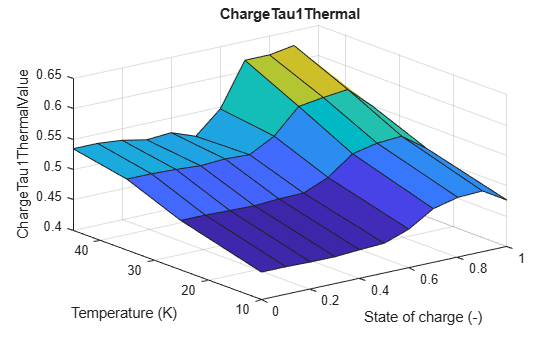```figure('Name','Voltage profile for original and parameterized cell'); plot(resDriveProfile.Time,resDriveProfile.V); title('Voltage (V) for Original and Parameterized Cell') xlabel('Time (s)'); ylabel('Voltage (V)'); legend('Original Cell', 'Parameterized Cell')```If the error is not within acceptable limits, try with a different initial guess, a different number of RC pairs, or by using a different fitting method (fminsearch, Curve Fitting Toolbox).

If you want to characterize the battery at multiple temperatures, use this workflow to fit the parameters at each individual temperature and then combine them inside the temperature dependent block.

### Reference

1. Christophersen, Jon P. Battery Test Manual For Electric Vehicles, Revision 3. United States: N. p., 2015. Web. doi:10.2172/1186745